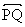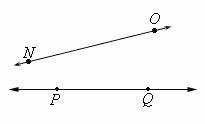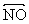Definition Of Line

A Line is a straight path that is endless in both directions.

The representation for the line PQ is.
A line does not have any thickness.

Example of LineIn the above figure, NO and PQ extend endlessly in both directions. So,andrepresent lines.

Solved Example on Line

Ques: Identify a figure that can be called a line.A. Figure 4
B. Figure 2
C. Figure 1
D. Figure 3